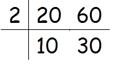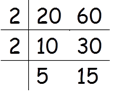This method is to find LCM of given numbers.

Let’s say we want to find LCM of 20 and 60.

First write 4 and 20 as shown belowDivide 20 and 60 by 2Divide 10 and 30 by 2We can not divide any of 5 and 15 by 2 perfectly. So divide them by three.

Here 5 is not divisible by 3 so 5 is written as it is.Divide 5 and 5 by 5.Stop this process when last row has all 1s.

So the LCM is 2 × 2 × 3 × 5 = 60